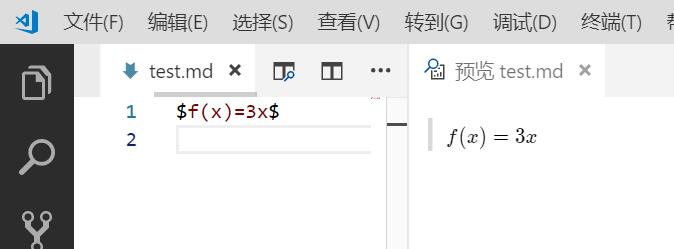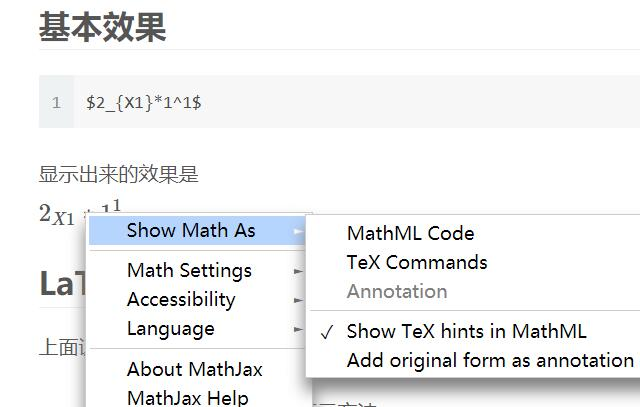0%

# MathJax简介

MathJax是一款运行在浏览器中的开源的数学符号渲染引擎，使用MathJax可以方便的在浏览器中显示数学公式，不需要使用图片。目前，MathJax可以解析Latex、MathML和ASCIIMathML的标记语言。

https://mathpix.com/

# VScode# Gitbook

Gitbook官方插件地址 -> https://plugins.gitbook.com/

Gitbook里面当然也有对应的插件了。有两种插件katexmathjax，我还是先选择了mathjax。在官网上katex上写的是比mathjax更快，但是我选择mathjax还有的一个点是因为可以在网页上右键非常方便得显示出MathML格式的代码和LaTeX格式的代码。

# 基本效果

$2_{X1}*1^1$# LaTeX公式书写

## 上标与下标

• 上标和下标分别使用^与_
• 例如x_i^2显示的效果就是$x_i^2$
• 使用大括号{}括起来表示一个单元，同时可以消除二义性

## 括号

• 小括号与方括号： 使用原始的()，[]即可，如(2+3)[4+4]的显示效果就是$(2+3)[4+4]$
• 大括号： 由于大括号{}被用来分组，因此需要使用\{ 和 \}表示大括号，也可以使用\lbrace\rbrace来表示。如\{a*b\}的显示效果为${ab}$，\lbrace ab \rbrace的效果也为$\lbrace a*b \rbrace$。
• 尖括号：使用\langle\rangle表示左尖括号和右尖括号。如\langle x \rangle将表示为$\langle x \rangle$。
• 上取整：使用\lceil\rceil表示。 如，\lceil x \rceil：$\lceil x \rceil$。
• 下取整：使用\lfloor\rfloor表示。如，\lfloor x \rfloor：$\lfloor x \rfloor$。
• 不可见括号：使用.表示。
• 需要注意的是，原始符号并不会随着公式大小缩放。如，(\frac12)：$(\frac12)$。可以使用\left( … \right)(\left\lbrave...\right\rbrace,可以理解成\left,\right是对括号的修饰词)来自适应的调整括号大小。如下，

$$\lbrace \sum_{i=0}^n i^2 = \frac{(n^2+n)(2n+1)}{6}\rbrace \tag{1.1}$$

$$\left\lbrace \sum_{i=0}^n i^2 = \frac{(n^2+n)(2n+1)}{6}\right\rbrace \tag{1.2}$$

## 求和与积分

• \sum用来表示求和符号，其下标表示求和下限，上标表示上限。如，\sum_1^n：$\sum_1^n$。
• \int用来表示积分符号，同样地，其上下标表示积分的上下限。如，\int_1^\infty：$\int_1^\infty$。
• 与此类似的符号还有，\prod：$\prod$，\bigcup:$\bigcup$，\bigcap：$\bigcap$，\iint：$\iint$。

## 分式与根式

• 分式的表示。第一种，使用\frac ab\frac作用于其后的两个组a，b，结果为$\frac ab$。如果你的分子或分母不是单个字符，请使用{..}来分组。第二种，使用\over来分隔一个组的前后两部分，如{a+1\over b+1}：${a+1\over b+1}$。
• 根式使用\sqrt来表示。如，\sqrt {\frac xy}：$\sqrt {\frac xy}$

## 字体

• 使用\mathbb或\Bbb显示黑板粗体字，此字体经常用来表示代表实数、整数、有理数、复数的大写字母。如，

$$\mathbb{CHNQRZ}$$

• 使用\mathbf显示黑体字，如

$$\mathbf{ABCDEFGHIJKLMNOPQRSTUVWXYZ}$$
$$\mathbf{abcdefghijklmnopqrstuvwxyz}$$

• 使用\mathtt显示打印机字体，如

$$\mathtt{ABCDEFGHIJKLMNOPQRSTUVWXYZ}$$
$$\mathtt{abcdefghijklmnopqrstuvwxyz}$$

• 使用\mathrm显示罗马字体，如

$$\mathrm{ABCDEFGHIJKLMNOPQRSTUVWXYZ}$$
$$\mathrm{abcdefghijklmnopqrstuvwxyz}$$

• 使用\mathscr显示手写体,如

$$\mathscr{ABCDEFGHIJKLMNOPQRSTUVWXYZ}$$

• 使用\mathfrak显示Fraktur字母（一种德国字体），

$$\mathfrak{ABCDEFGHIJKLMNOPQRSTUVWXYZ}$$
$$\mathfrak{abcdefghijklmnopqrstuvwxyz}$$

## 特殊函数与符号

• 常见的三角函数，求极限符号可直接使用+缩写即可。如\sin x表示为$\sin x$,\arctan表示为$\arctan$,lim_{1\to\infty}表示为$lim_{1\to\infty}$。
• 比较运算符：\lt \gt \le \ge \neq ：$\lt \gt \le \ge \neq$。
• 可以在这些运算符前面加上\not，如\not\lt：$\not\lt$。
• \times \div \pm \mp表示：$\times \div \pm \mp$，\cdot表示居中的点$\cdot$，x \cdot y : $x \cdot y$。
• 集合关系与运算：\cup \cap \setminus \subset \subseteq \subsetneq \supset \in \notin \emptyset \varnothing ：$\cup \cap \setminus \subset \subseteq \subsetneq \supset \in \notin \emptyset \varnothing$.
• 表示排列使用{n+1 \choose 2k}\binom{n+1}{2k}，$\binom{n+1}{2k}$。
• 箭头：\to \rightarrow \leftarrow \Rightarrow \Leftarrow \mapsto : $\to \rightarrow \leftarrow \Rightarrow \Leftarrow \mapsto$。
• 逻辑运算符：\land \lor \lnot \forall \exists \top \bot \vdash \vDash ：$\land \lor \lnot \forall \exists \top \bot \vdash \vDash$。
• \star \ast \oplus \circ \bullet ：$\star \ast \oplus \circ \bullet$。
• \approx \sim \cong \equiv \prec ：$\approx \sim \cong \equiv \prec$。
• \infty \aleph_0 $\infty \aleph_0$ \nabla \partial $\nabla \partial$ \Im \Re: $\Im \Re$。
• 模运算 \pmode, 如，a\equiv b\pmod n：$a\equiv b\pmod n$。
• \ldots与\cdots，其区别是dots的位置不同，ldots位置稍低，cdots位置居中。$a_1+a_2+\cdots+a_n$，$a_1, a_2, \ldots ,a_n$。
• 一些希腊字母具有变体形式，如 \epsilon \varepsilon : $\epsilon \varepsilon$, \phi \varphi: $\phi \varphi$。